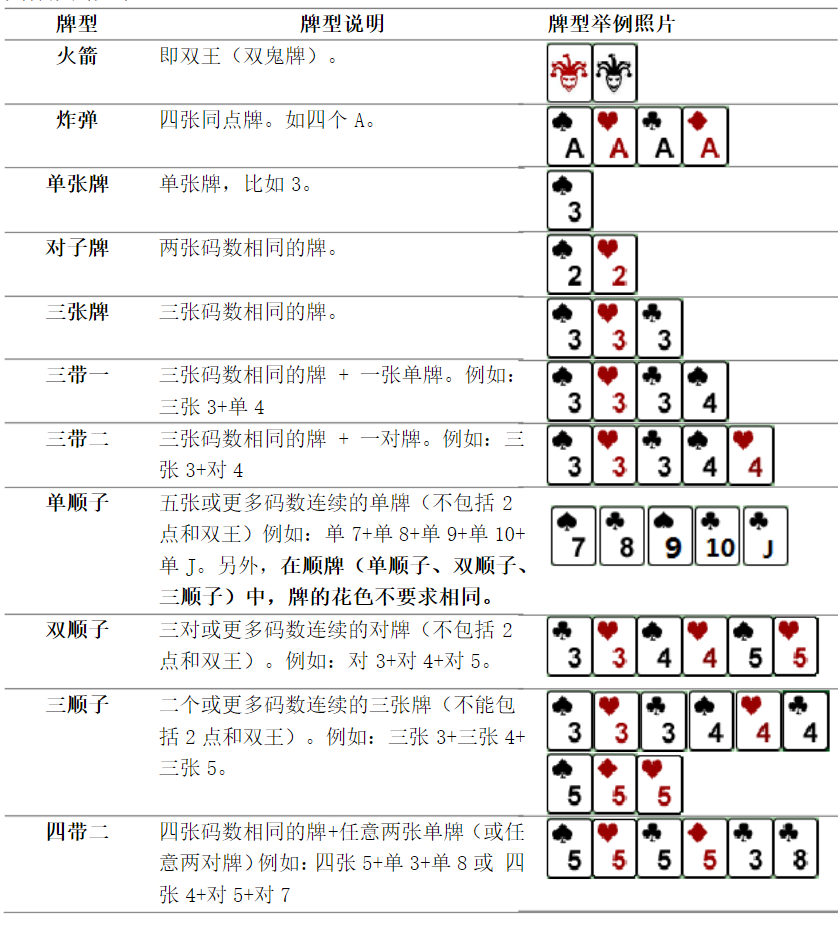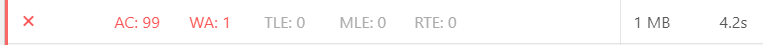# 洛谷 P2668 NOIp2015提高组 斗地主 题解【模拟】【搜索】【估价】

十分毒瘤的枚举题。

## 题目描述## 输入输出格式

$Q$

，13表示数码K；黑桃、红心、梅花、方片分别用1-4来表示；小王的表示方法为0 1，大王的表示方法为0 2。

## 输入输出样例

1 8
7 4
8 4
9 1
10 4
11 1
5 1
1 4
1 1

3


1 17
12 3
4 3
2 3
5 4
10 2
3 3
12 2
0 1
1 3
10 1
6 2
12 1
11 3
5 2
12 4
2 2
7 2


6


## 说明

$1$

组手牌，包含8张牌：方片

$7$

，方片

$8$

，黑桃

$9$

，方片

$10$

，黑桃

$J$

，黑桃

$5$

，方片

$A$

以及黑桃

$A$

。可以通过打单顺子（方片

$7$

，方片

$8$

，黑桃

$9$

，方片

$10$

，黑桃

$J$

），单张牌（黑桃

$5$

）以及对子牌（黑桃

$A$

以及方片

$A$

）在

$3$

次内打光。

$T$

与张数

$n$

的规模如下：

### n

1 100 2 11 100 14
2 100 2 12 100 15
3 100 3 13 10 16
4 100 3 14 10 17
5 100 4 15 10 18
6 100 4 16 10 19
7 100 10 17 10 20
8 100 11 18 10 21
9 100 12 19 10 22
10 100 13 20 10 23

## 题解：

这种题目意思一清二楚的题当然不是在考什么算法，而是代码能力和剪枝技巧。对于这种$latex n\le 23$的数据，就可以考虑枚举了。

因为在一副手牌中，三带一等特殊牌型出现的次数不会特别多，而顺子又特别灵活，所以我们首先枚举顺子（有时为了顺子而拆对牌或特殊牌型会更优）。对于一串长为$latex len\ge 5$的顺子来说，它所包含的子顺子有$latex \frac{(len-4)(len-3)}2$个，我们要保证这些都被枚举到。

接下来我们如果先去搜索条件更严苛的，应该会搜得更快，除了顺子以外的牌型，根据牌数大小递减枚举可行解，进行深搜。

除了上述的剪枝，我们还要加入最优性剪枝。因为在枚举完顺子后，我们让牌型大小单调递减，因此如果剩下的牌数(记为r)和当前牌型大小(记为n)的商$latex \lfloor \frac rn \rfloor$加上当前步数已经大于搜过的最优解了，这个状态就可以被舍弃了。这个$latex \lfloor \frac rn \rfloor$可以被视作一个估价函数，方便剪枝。

还有一点要注意的就是，单独的炸弹可以被看作一个三带一，就不用多枚举一个炸弹的函数入口了，多一个入口就会把三带一后面的状态又重新枚举一遍，这样浪费了很多时间。

vijos上到现在还是AC99WA1不知道为什么qaq。。。luogu增强版有两个点要判一下相邻两点输入是否完全一样（相当于打表了。。。）才能过，不知道是哪里剪枝不够完美。

dfs回溯时一定要把所有状态清零。

## Code：

#include<cstdio>
#include<cstring>
int cd,n,num;
int sum=0,ans=100000000;
void conti(int x);
void four(int x);
void three(int x);
void two();
void dfs()
{
sum++;
if(num==0)
{
ans=ans<sum?ans:sum;
sum--;
return;
}
conti(3);//三顺
conti(2);//双顺
conti(1);//单顺
four(2);//四带两对
four(1);//四带两张
three(2);//三带二
three(1);//三带一
two();//对子
ans=ans<sum+num?ans:sum+num;//剩下全当单牌
sum--;
return;
}
int len={0,5,3,1};
int main()
{
//freopen("testdata.in","r",stdin);
int T;
scanf("%d%d",&T,&n);
num=n;
while(T--)
{
int u,v;
memset(cd,0,sizeof(cd));
for(int i=1;i<=n;i++)
{
scanf("%d%d",&u,&v);
cd[u]++;
}
sum=-1,ans=100000000;
dfs();
//printf("%d\n",sum);
printf("%d\n",ans);
}
}
void conti(int x)
{
int tt=0;
for(int i=3;i<=13;i++)
{
if(cd[i]>=x)
tt++;
else
tt=0;
if(tt>=len[x])
{
int tmp=tt;
while(tmp>=len[x])
{
for(int j=i;j>i-tmp;j--)
{
cd[j]-=x;
num-=x;
}
if(sum+num/(tmp*x)<ans-1)
dfs();
for(int j=i;j>i-tmp;j--)
{
cd[j]+=x;
num+=x;
}
tmp--;
}
}
}
if(tt>=len[x]-1&&cd>=x)
{
tt++;
while(tt>=len[x])
{
for(int j=13;j>13-tt+1;j--)
{
cd[j]-=x;
num-=x;
}
num-=x;
cd-=x;
if(sum+num/(tt*x)<ans-1)
dfs();
cd+=x;
num+=x;
for(int j=13;j>13-tt+1;j--)
{
cd[j]+=x;
num+=x;
}
tt--;
}
}
}

void four(int x)
{
num-=4+2*x;
for(int i=13;i>=1;i--)//四带几
if(cd[i]>=4)
{
cd[i]-=4;
for(int j=0;j<=13;j++)
if(cd[j]>=x)
{
cd[j]-=x;
for(int k=j;k<=13;k++)
if(cd[k]>=x)
{
cd[k]-=x;
if(sum+num/(4+2*x)<ans-1)
dfs();
cd[k]+=x;
}
cd[j]+=x;
}
cd[i]+=4;
}
num+=4+2*x;
}

void three(int x)
{
num-=3+x;
for(int i=13;i>=1;i--)
if(cd[i]>=3)
{
cd[i]-=3;
for(int j=0;j<=13;j++)
if(cd[j]>=x)
{
cd[j]-=x;
if(sum+num/(3+x)<ans-1)
dfs();
cd[j]+=x;
}
cd[i]+=3;
}
num+=3+x;
}

void two()
{
num-=2;
for(int i=0;i<=13;i++)
if(cd[i]>=2)
{
cd[i]-=2;
if(sum+num/2<ans-1)
dfs();
cd[i]+=2;
}
num+=2;
}


### 说点什么Subscribe

/* */